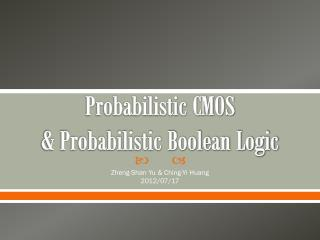DownloadDownload PresentationProbabilistic CMOS & Probabilistic Boolean Logic

# Probabilistic CMOS & Probabilistic Boolean Logic

Download Presentation## Probabilistic CMOS & Probabilistic Boolean Logic

- - - - - - - - - - - - - - - - - - - - - - - - - - - E N D - - - - - - - - - - - - - - - - - - - - - - - - - - -
##### Presentation Transcript

1. Probabilistic CMOS & Probabilistic Boolean Logic Zheng-Shan Yu & Ching-Yi Huang 2012/07/17

2. Outline • PCMOS • CMOS with noise • CMOS with varying voltages • Correctness v.s. energy • Relations between PBF, PBTT, and PBC • PBL optimization • Problem • Future work

3. CMOS with noise • NOT:

4. CMOS with noise (cont.) • Noise: • Interconnection noise • Crosstalk • Simultaneous switching noise • Thermal noise • Noise models of PCMOS:

5. CMOS with varying voltages • Many voltage regulators are required if an application requires many different probabilities. • It is much better that the number of probabilities is small. With noise Without noise

6. Correctness v.s. energy • It is much better that the number of probabilistic gates is large.

7. Relations between PBF, PBTT, and PBC (1/5) • ((A∧B)∨(B∧C))∨3/4(A∧C) PBF PBC PBTT

8. Relations between PBF, PBTT, and PBC (2/5) • ((A∧B)∨(B∧C))∨3/4(A∧C) PBF PBC

9. Relations between PBF, PBTT, and PBC (3/5) • o1 = (n1 ∧4/5 n1) • n1 = (~2/3a) • o1=((~2/3a)∧4/5(~2/3a)) PBF PBC with reconvergentfanout o1 n1

10. Relations between PBF, PBTT, and PBC (4/5) • ((A∧B)∨(B∧C))∨3/4(A∧C) PBF • NOT: • Pout = (1 – Pin)*p + Pin*(1 – p) • OR: • Pout = (1 – Pa)*(1 – Pb)*(1 – p) + (1 – Pa)*Pb*p + Pa*(1 – Pb)*p + Pa*Pb*p • AND: • Pout = (1 – Pa)*(1 – Pb)*(1 – p) + (1 – Pa)*Pb*(1 – p) + Pa*(1 – Pb)*(1 – p) + Pa*Pb*p PBTT

11. Relations between PBF, PBTT, and PBC (5/5) • ((A∧B)∨(B∧C))∨3/4(A∧C) PBF PBTT

12. PBL optimization (1/8) • (A ∧ 3/4 B) • ( (A’B’)’)∧ (A’B’) + ( (A’B)’)∧ (A’B) + ( (AB’)’)∧ (AB’) + ( (AB)’)∧ (AB) A B

13. PBL optimization (2/8) • Associativity: • ((A∧ pB)∧pC) ≠ (A ∧ p(B ∧pC)) • Distributivity: • (A∧ r B)∨p(A∧rC) ≠ A ∧ r(B ∨pC)

14. PBL optimization (3/8) • Step1: Optimize deterministic cubes • ((A’B’ + A’B + AB’)’)∧ (A’B’ + A’B + AB’)) => (((AB)’)’)∧ ((AB)’) • ( (AB)’)∧ (AB)

15. PBL optimization (4/8) • Step2: Re-arrange probabilities • (((AB)’)’)∧ ((AB)’) • => ((AB)’)∧ ((AB)’) • ( (AB)’)∧ (AB)

16. PBL optimization (5/8) • Step3: Merge probabilistic cubes • ((AB)’)∧ ((AB)’) • ( (AB)’)∧ (AB) • Combine : • ( (AB)’)∧ ((AB)’ + AB) • => ( (AB)’) • => (((AB))) • => (((A ∧ 3/4 B))) • Goal: (A ∧ 3/4 B)

17. PBL optimization (6/8) • Step1: Optimize deterministic cubes • Conventional logic optimization • Step2: Re-arrange probabilities 1 =pq+(1-p)(1-q)

18. PBL optimization (7/8) • Step3: Merge probabilistic cubes • ( (AB)’)∧ ((AB)’) • ( (AB)’)∧ (AB) Mutually exclusive A B A B A B

19. PBL optimization (8/8) • Step3: Merge probabilistic cubes • ( (AB)’)∧ ((AB)’) • ( (AB)’)∧ (AB) • ( (AB)’)∧ ((AB)’ ∨AB) A B A B

20. Problem • PBL optimization

21. Future work • Assign probabilistic gates for error-tolerant designs • Testibility • Observability • Controllability

22. Future work (cont.) • Compute the output probability under an input assignment • Monte Carlo Simulation • Deterministic methods